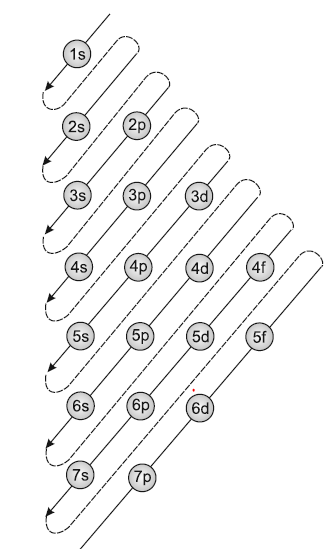# Aufbau Principle

## Aufbau rule or Principle

• The word 'aufbau' in German means 'building up'. The building up of orbitals means the filling up of orbitals with electrons
• It states that in ground state of atom, the orbitals are filled in increasing order of their energies.
• So electrons first occupy the lowest energy orbital available to them and enter into higher energy orbitals only after the lower energy orbitals are filled as per Pauli Exclusion principle & Hund Rule
• Now we know that energy of a given orbital depends upon effective nuclear charge and different type of orbitals are affected to different extent. So there is no single ordering of energies of orbitals which will be universally correct for all atoms
• But Following ordering can be used to predict the configuration of electrons in atom
1s, 2s ,2p, 3s ,3p ,4s ,3d ,4p, 5s,4d,5p,4f,5d,6p

This can be remembered with below diagram• The above order is based on Madulung Rule which states that a.The sum of the values of the principal quantum numbers (n) and azimuthal quantum number (l) i.e (n+l) determined the energy level of an orbital.Subshell having lower n+l value will be filled first
b.The lower value of the sum of (n+l) implies that the energy of the orbital is low. If the value of n+l for two orbitals is equal then the orbital with a lower value of n will have a lower energy level.

• Question 1
In a given atom no two electrons can have the same values for all the four quantum numbers. This is called
(a) Hund's rule
(b) Aufbau's principle
(c) Uncertainty principle
(d) Pauli's exclusion principle

Solution
(d)

Question 2
The arrangement of orbitals on the basis of energy is based upon their (n+l ) value. Lower the value of (n+l ), lower is the energy. For orbitals having same values of (n+l), the orbital with lower value of n will have lower energy.
I. Based upon the above information, arrange the following orbitals in the increasing order of energy.
(a) 1s, 2s, 3s, 2p
(b) 4s, 3s, 3p, 4d
(c) 5p, 4d, 5d, 4f, 6s
(d) 5f, 6d, 7s, 7p
II. Based upon the above information, solve the questions given below :
(a) Which of the following orbitals has the lowest energy?
4d, 4f, 5s, 5p
(b) Which of the following orbitals has the highest energy?
5p, 5d, 5f, 6s, 6p
Solution
I.
(a) 1s < 2s < 2p < 3s
(b) 3s < 3p < 4s < 4d
(c) 4d < 5p < 6s < 4f < 5d
(d) 7s < 5f < 6d < 7p
II. (a) 5s (b) 5f

Question 3
When 3d orbital is complete, the new electron will enter the
(a) 4p-orbital
(b) 4f-orbital
(d) 4s-orbital
(d) 4d-orbital
Solution
(a)